# The robust sandwich variance estimator for linear regression (theory)

In a previous post we looked at the properties of the ordinary least squares linear regression estimator when the covariates, as well as the outcome, are considered as random variables. In this post we’ll look at the theory sandwich (sometimes called robust) variance estimator for linear regression. See this post for details on how to use the sandwich variance estimator in R.

In the earlier post we assumed that, where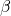is a column vector of regression coefficients to be estimated.

Using estimating equation theory, we showed that the estimator has variance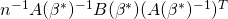where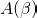denotes the matrix is equal to minus the derivative of the estimating function with respect to the parameter,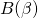denotes the variance covariance matrix of the estimating function, and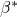denotes the true value of. We then found expressions for these population quantities, and estimators for them. We found that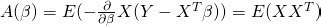and that this could be estimated by its empirical mean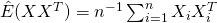The matrixwas given as the variance of the estimating function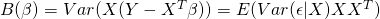In the previous post, we then derived an expression for this assuming that the residuals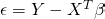have constant variance (as the covariates vary). Here we will relax that assumption, such thatmay have a variance that varies with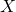. In this case, how might we estimate? Since by assumption the residuals have mean zero conditional on, if subjecthas predictor values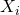, remembering that the variance is the average squared deviation around the mean, we can estimate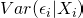by the square of the estimated residual residual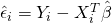, i.e.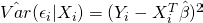The matrixcan then be estimated by taking the average of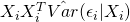across the sample, substituting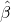in place of the unknown (true) value: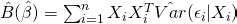An alternative route to getting to the same estimator is to directly estimate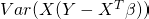by its sample variance, and using the fact that the this estimating function has mean zero.

The variance estimator we have derived here is consistent irrespective of whether the residuals in the regression model have constant variance. It is called the sandwich variance estimator because of its form in which the B matrix is sandwiched between the inverse of the A matrix.

When we suspect, or find evidence on the basis of a test for heteroscedascity, that the variance is not constant, the standard OLS variance should not be used since it gives biased estimate of precision. A by-product of this is that p-values for hypothesis tests and confidence intervals, which use the estimated variance, will not perform as they should – the type I error rate may not be correct, and the coverage rate of the confidence intervals will in general not meet their nominal level.

In this case, the sandwich estimator we have derived here can be used. This is sometimes called the robust estimator of variance, since it is robust to non-constant residual variance. An alternative to using the robust sandwich variance estimator would be to use bootstrapping.

For more details on the robust sandwich variance estimator, and semiparametric methods more generally, I recommend Tsiatis’ book ’Semiparametric Theory and Missing Data’.

### 1 thought on “The robust sandwich variance estimator for linear regression (theory)”

1.Someone asks: The sandwich estimator is known to be “heteroskedasticity-consistent”, but I have read that it is “nonlinearity-consistent” as well. OLS regression is much easier to interpret than generalized additive model (GAM).
So, in case of nonlinearities in the regression function and in the regressors, why (in which situations) should we use/prefer generalized additive model (GAM) instead of classical OLS with sandwich estimator?

My answer: I’m not 100% sure what is meant by nonlinearity consistent here. Suppose you model E(Y|X)=a+b*X, and estimate a and b using OLS. Then without any assumptions they are unbiased for the ‘parameters’ a and b that represent the best linear approximation to the conditional mean function. Sandwich variances will then give you valid frequentist variance estimates for the estimates of a and b. But if E(Y|X) is not linear in X, you are not getting the true dependence of the mean of Y on X.

In a GAM, you can more flexibly model how E(Y|X) depends on X (i.e. with complicated non-linear functions). If you care about the nature of this dependency, then you should be concerned with correctly modelling it, and so GAMs may be a nice approach.